• 今天小编就为大家分享一篇Python turtle图库&&姓名实例，具有很好的参考价值，希望对大家有所帮助。一起跟随小编过来看看吧
• 班里大神用python是这么的，果然只有对比才有伤害，，，（为什么大神说打开画图就可以看坐标画图，我一点都不会啊啊啊啊），所以只能惨烈的一点点修改，因为太丑了所以记录一下吧，万一以后会大神的方法了呢?...
       班里大神用python是这么画的，果然只有对比才有伤害，，，（为什么大神说打开画图就可以看坐标画图，我一点都不会啊啊啊啊），所以只能惨烈的一点点修改，因为太丑了所以记录一下吧，万一以后会大神的方法了呢?

先把大神的链接放出来对比一下，不要放了就都走了啊啊啊啊
https://blog.todest.cn/post/50bbd1a0/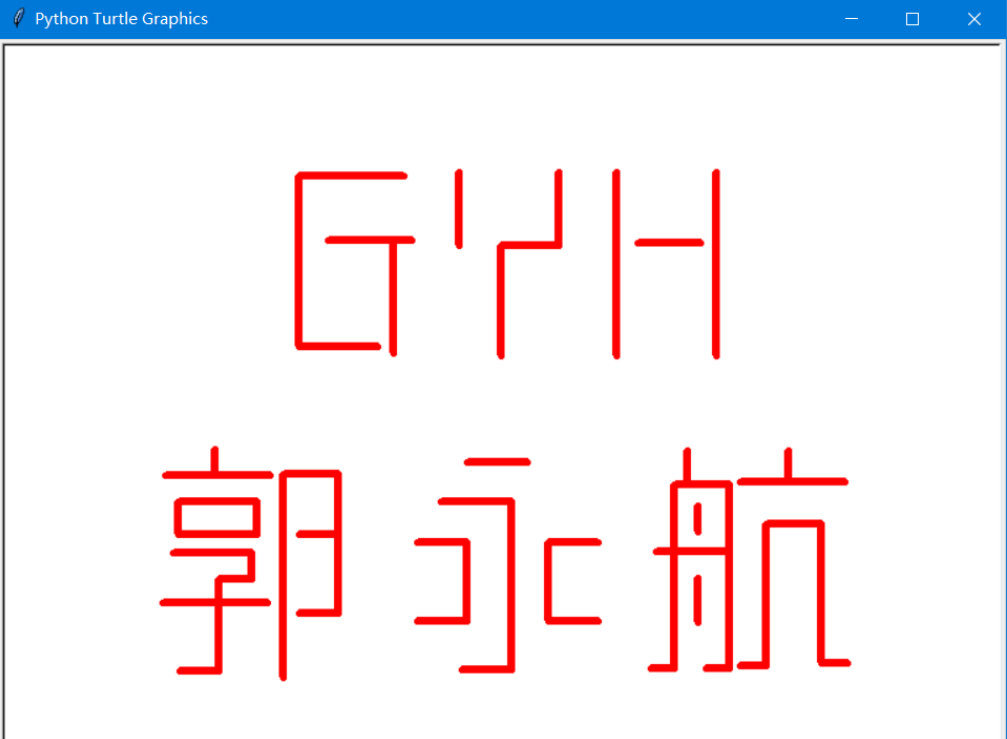好了，放自己的了，明明代码长度差不多！！！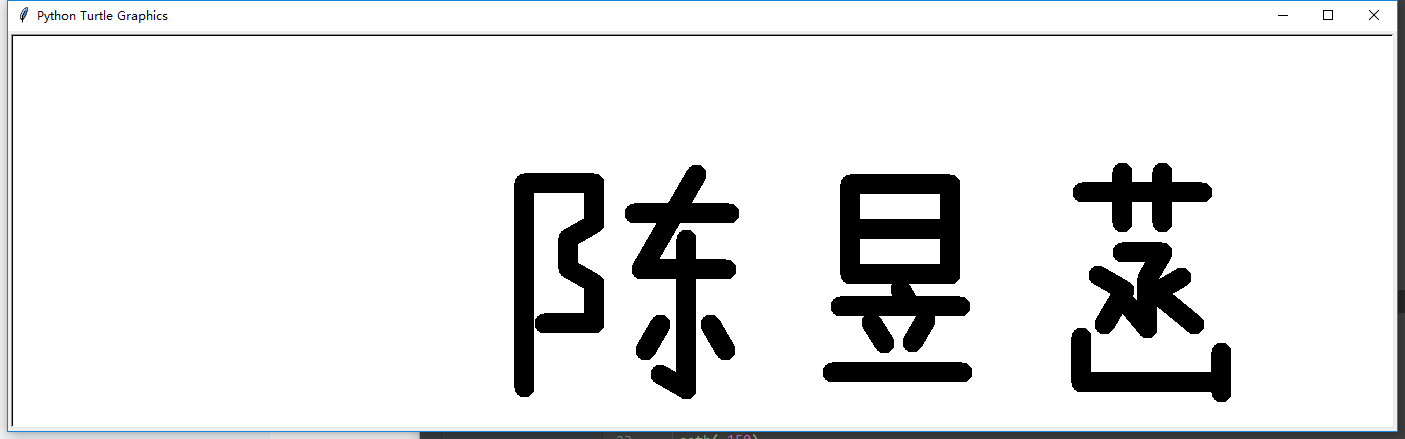不丢人了，溜了溜了，，
from turtle import *
setup(1200,400,200,200)

#陈
penup()
fd(-181)
seth(90)
fd(51)
seth(-90)
pendown()
pensize(20)
fd(204)

penup()
seth(90)
fd(204)
seth(0)
pendown()
fd(70)

seth(-90)
fd(40)
seth(-150)
fd(30)
seth(-90)
fd(30)
seth(-30)
fd(30)
seth(-90)
fd(40)
seth(180)
fd(50)

penup()
seth(90)
fd(110)
seth(0)
fd(90)
pendown()

fd(95)
penup()
seth(180)
fd(40)
seth(80)
fd(40)
pendown()

seth(-120)
fd(110)
seth(0)
fd(85)
penup()
seth(180)
fd(40)
seth(90)
fd(30)
seth(-90)
pendown()
fd(150)
seth(150)
fd(30)
penup()
seth(90)
fd(50)
pendown()
seth(-120)
fd(30)

penup()
seth(0)
fd(80)
pendown()
seth(120)
fd(30)

#昱
penup()
seth(0)
fd(140)
seth(90)
fd(140)
pendown()
seth(-90)
fd(90)
seth(0)
fd(100)
seth(90)
fd(90)
seth(180)
fd(100)
seth(-90)
fd(45)
seth(0)
fd(100)

penup()
seth(-90)
fd(60)
seth(180)
fd(50)
pendown()
seth(-60)
fd(20)
penup()
seth(180)
fd(70)
pendown()
seth(0)
fd(120)
penup()
seth(-170)
fd(90)
pendown()
seth(-60)
fd(25)
penup()
seth(30)
fd(47)
pendown()
seth(-120)
fd(25)
penup()
seth(-90)
fd(30)
seth(180)
fd(80)
pendown()
seth(0)
fd(130)

#菡
penup()
seth(0)
fd(120)
seth(90)
fd(180)
pendown()
seth(0)
fd(120)
penup()
seth(180)
fd(80)
seth(90)
fd(20)
pendown()
seth(-90)
fd(50)
penup()
seth(90)
fd(50)
seth(0)
fd(40)
pendown()
seth(-90)
fd(50)

penup()
fd(30)
seth(180)
fd(40)
pendown()
seth(0)
fd(40)

seth(-120)
fd(30)
seth(-90)
fd(50)
seth(130)
fd(30)

penup()
seth(90)
fd(30)
seth(180)
fd(30)
pendown()

seth(-30)
fd(30)
seth(-120)
fd(40)

penup()
seth(0)
fd(90)
pendown()

seth(140)
fd(50)
seth(30)
fd(30)

penup()
seth(-90)
fd(60)
seth(-180)
fd(100)
pendown()

seth(-90)
fd(45)
seth(0)
fd(140)
seth(90)
fd(30)
seth(-90)
fd(40)

done()



展开全文• ## Python画名字

千次阅读 2020-03-24 10:37:50
import turtle # 其实，这个库可以按照英文理解成脚下抹了颜料的小海龟 def run(angle, length): # 自定义的run函数 turtle.seth(angle) # seth(angle)是海龟在平面直角坐标系爬行的角度 turtle.fd(length) ...
import turtle
# 其实，这个库可以按照英文理解成脚下抹了颜料的小海龟
def run(angle, length):
# 自定义的run函数
turtle.seth(angle)
# seth(angle)是海龟在平面直角坐标系爬行的角度
turtle.fd(length)
# fd(length)是它爬行的距离

def locate(x, y):
# 自定义的定位函数：把海龟拿到一个坐标（x，y）上
turtle.penup()
# 拿起海龟
turtle.goto(x, y)
# 拿到坐标（x,y）
turtle.pendown()
# 放下海龟(开始爬出图形)

def init():
turtle.setup(800, 400, 200, 200)
# 开一个窗口，具体参数参见书本
turtle.pensize(10)
# 图形线条的粗细
turtle.pencolor("black")
# 线条的颜色

init()

# 刘
#坐标
locate(-310,170)
#方向和爬行长度
run(-90,35)
locate(-360,135)
run(0,100)
locate(-280,135)
run(-125,120)
locate(-340,135)
run(-55,120)
locate(-220,155)
run(-90,120)
locate(-180,165)
run(-90,160)
locate(-180,5)
run(135,35)
# 边
locate(-115,175)
run(-115,25)
locate(-160,150)
run(0,80)
locate(-80,150)
run(-135,80)
locate(-140,92)
run(-25,70)
locate(-70,60)
run(-135,100)
locate(-150,00)
run(0,200)
locate(-10,165)
run(-110,120)
locate(-60,120)
run(0,85)
locate(25,120)
run(-90,70)
locate(25,50)
run(135,25)
#防
locate(100,175)
run(-90,180)
locate(100,175)
run(0,45)
locate(145,175)
run(-127,75)
locate(100,115)
run(0,45)
locate(145,115)
run(-127,75)
locate(280,175)
run(-150,20)
locate(180,159)
run(0,160)
locate(230,159)
run(-115,170)
locate(210,109)
run(0,100)
locate(315,109)
run(-115,120)
locate(260,2)
run(165,30)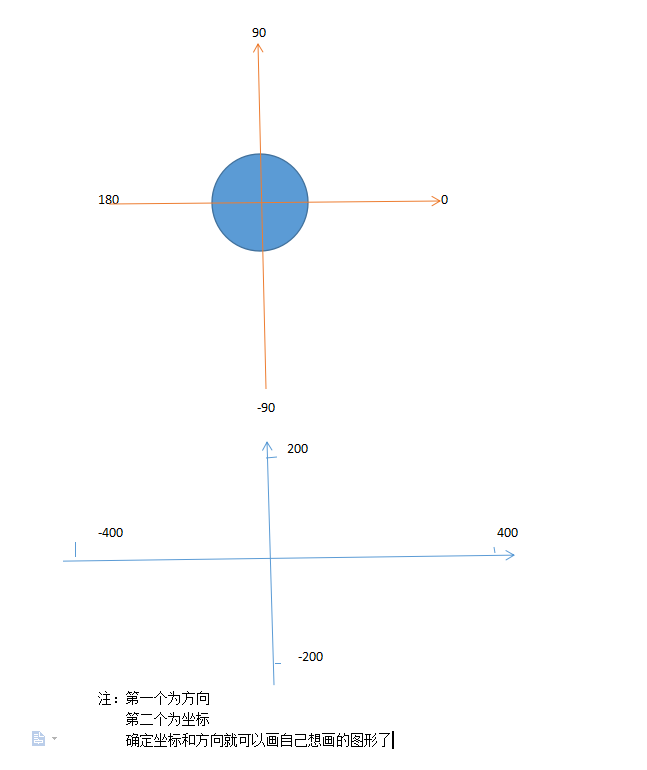展开全文• 一个简单的Python绘图案例。通过Python的turtle库来绘制一个爱心。
• Turtle库是Python语言中一个很流行的绘制...这个动图展示的就是我利用Turtle库画的一个名字画，下面附上代码： import time import turtle from random import random t = turtle t.speed(3) t.hideturtle() t.color
Turtle库是Python语言中一个很流行的绘制图像的函数库，利用这个库会生成一个画布，在画布中有我们看不见的一个默认以中心点为原点的坐标轴，在原点会有一个初始默认朝东的画笔（箭头），一般我们把这个箭头叫做海龟，箭头的朝向就是海龟头的朝向。这个动图展示的就是我利用Turtle库画的一个名字画，下面附上代码：
import time
import turtle
from random import random

t = turtle
t.speed(3)
t.hideturtle()
t.color('black')
t.pensize(10)

# 一
t.penup()
t.goto(-110, 60)
t.pendown()
t.forward(100)

# |
t.penup()
t.goto(-60, 125)
t.right(90)
t.pendown()
t.forward(266)

# 丿
t.penup()
t.goto(-60, 45)
t.right(45)
t.pendown()
t.forward(90)

# 丶
t.penup()
t.goto(-60, 38)
t.left(90)
t.pendown()
t.forward(40)

# 小一
t.penup()
t.goto(20, 60)
t.left(45)
t.pendown()
t.forward(90)

# |
t.penup()
t.goto(65, 123)
t.right(90)
t.pendown()
t.forward(123)

# 大一
t.penup()
t.goto(0, -5)
t.left(90)
t.pendown()
t.forward(130)

# 小一
t.penup()
t.goto(20, -65)
t.pendown()
t.forward(90)

# |
t.penup()
t.goto(65, -14)
t.right(90)
t.pendown()
t.forward(120)

# 大一
t.penup()
t.goto(0, -135)
t.left(90)
t.pendown()
t.forward(130)

# 画圆
t.color('red')
t.pensize(3)
t.penup()
t.goto(15, -200)
t.pendown()
t.circle(180)

t.exitonclick()


函数解释hideturtle隐藏画笔speed画笔速度，1-10，1最慢，10最快color后可传参（2个参数），不传为默认设置，第一个参数为画笔颜色，第二个参数为填充颜色pensize画笔粗细，越大越粗penup画笔拿起，之后画笔运动时不画下轨迹pendown默认是这个状态，画笔落下，之后画笔运动会画出轨迹forward（num）画笔运动，num为运动像素数，即长度right (a)画笔方向向右旋转a角度,顺时针旋转left(a)画笔方向向左旋转a角度,逆时针旋转goto(x,y)将画笔移动到坐标轴x,y位置，在pendowm下会画下轨迹goto(x,y)将画笔移动到坐标轴x,y位置，在pendowm下会画下轨迹circle(a)以当前点为圆心，画一个半径为a的圆exitonclick点击关闭，画笔运行完不再自动退出画布
下面是在论坛看到的一个大佬的画樱花树的代码，生成的结果比上面的复杂很多，但在弄懂我上面代码的情况下很容易看懂，作为进阶给大家展示下：import turtle as T
import random
import time

# 画樱花的躯干(60,t)
def Tree(branch, t):
time.sleep(0.0005)
if branch > 3:
if 8 <= branch <= 12:
if random.randint(0, 2) == 0:
t.color('snow')  # 白
else:
t.color('lightcoral')  # 淡珊瑚色
t.pensize(branch / 3)
elif branch < 8:
if random.randint(0, 1) == 0:
t.color('snow')
else:
t.color('lightcoral')  # 淡珊瑚色
t.pensize(branch / 2)
else:
t.color('sienna')  # 赭(zhě)色
t.pensize(branch / 10)  # 6
t.forward(branch)
a = 1.5 * random.random()
t.right(20 * a)
b = 1.5 * random.random()
Tree(branch - 10 * b, t)
t.left(40 * a)
Tree(branch - 10 * b, t)
t.right(20 * a)
t.up()
t.backward(branch)
t.down()

# 掉落的花瓣
def Petal(m, t):
for i in range(m):
a = 200 - 400 * random.random()
b = 10 - 20 * random.random()
t.up()
t.forward(b)
t.left(90)
t.forward(a)
t.down()
t.color('lightcoral')  # 淡珊瑚色
t.circle(1)
t.up()
t.backward(a)
t.right(90)
t.backward(b)

# 绘图区域
t = T.Turtle()
# 画布大小
w = T.Screen()
t.hideturtle()  # 隐藏画笔
t.getscreen().tracer(5, 0)
w.screensize(bg='wheat')  # wheat小麦
t.left(90)
t.up()
t.backward(150)
t.down()
t.color('sienna')

# 画樱花的躯干
Tree(60, t)
# 掉落的花瓣
Petal(200, t)
w.exitonclick()


这个代码除了用到我上面介绍的几个函数，还用到了screen和bgcolor，分别是设置画布和背景色,backward就是反向的forward。
好了，本次分享就到这里，大家喜欢或者觉得有用的话别忘了点赞收藏关注呀，下次见，拜拜

有问题的可以评论区或私信问我哦
展开全文turtle
• 利用python相关技术搭建的用户画像web轻量级应用 利用python相关技术搭建的用户画像web轻量级应用用户画像实现
• ## Python turtle画图库&&画姓名

万次阅读 多人点赞 2018-11-14 21:03:49
150） run(-90, 300)#从E点画到F点 画自己名字嘤嘤嘤 import turtle def run(angle, lenth): turtle.seth(angle) turtle.fd(lenth) def change(x, y): turtle.penup() turtle.goto(x, y) turtle.pendown() def ...

*****看一下我定义的change（）和run（）函数******

绘图坐标体系：
作用：设置主窗体的大小和位置
turtle.setup(width, height, startx, starty)

#width : 窗口宽度，若值是整数，表示像素值；若是小数，表示窗口宽度与屏幕的比例。#height ： 窗口高度，若值是整数，表示像素值；若是小数，表示窗口高度与屏幕的比例。#startx ： 窗口左侧与屏幕左侧的像素距离，若值是None，窗口位于屏幕水平中央。#starty ： 窗口顶部与屏幕顶部的像素距离，若值是None，窗口位于屏幕垂直中央。
画笔控制函数：
turtle中的画笔（即小海龟）可以通过一组函数来控制；
turtle.penup();                #抬起画笔（画笔移动时没有痕迹）
turtle.pendown();              #放下画笔（画笔移动时有痕迹，即作画中）
turtle.pensize(width)          #设置画笔的尺寸
turtle.width()                 #设置画笔宽度，当无参数输入时返回当前画笔宽度
turtle.pencolor(colorstring)   #设置画笔颜色
turtle.goto(x, y)              #将画笔移动至(x,y)处

形状绘制函数：
turtle.fd(distance)                    #画笔移动distance距离
turtle.seth(angle)                     #设置当前画笔的方向为angle
turtle.circle(radius, extent=None)     #根据半径radius绘制extent角度的弧形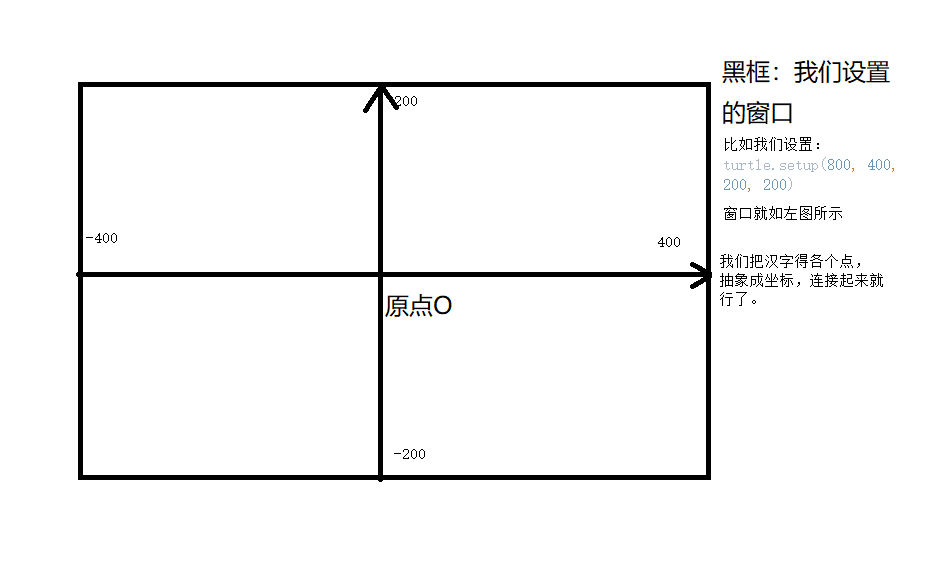我为了精简代码，定义了两个函数：
change(x, y) ： 将画笔的位置设置在直角坐标系中的 坐标(x, y)
run(angle,  length) ： 方向为angle ，画笔前进length个单位长度
def run(angle, lenth):
turtle.seth(angle)
turtle.fd(lenth)

def change(x, y):
turtle.penup()
turtle.goto(x, y)
turtle.pendown()

这里我使用 汉字 “中” 来举个例子：
我们把“中” 字抽象成点。（如图所示的 A、B、C、D、E、F 六个点）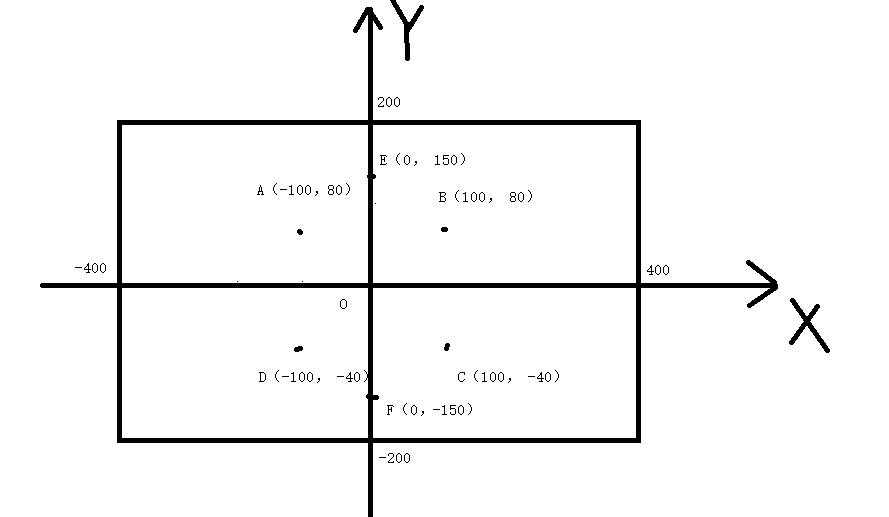然后，我们就是要把他们连接起来啊。 我们先连接ABCD四个点。
变成下图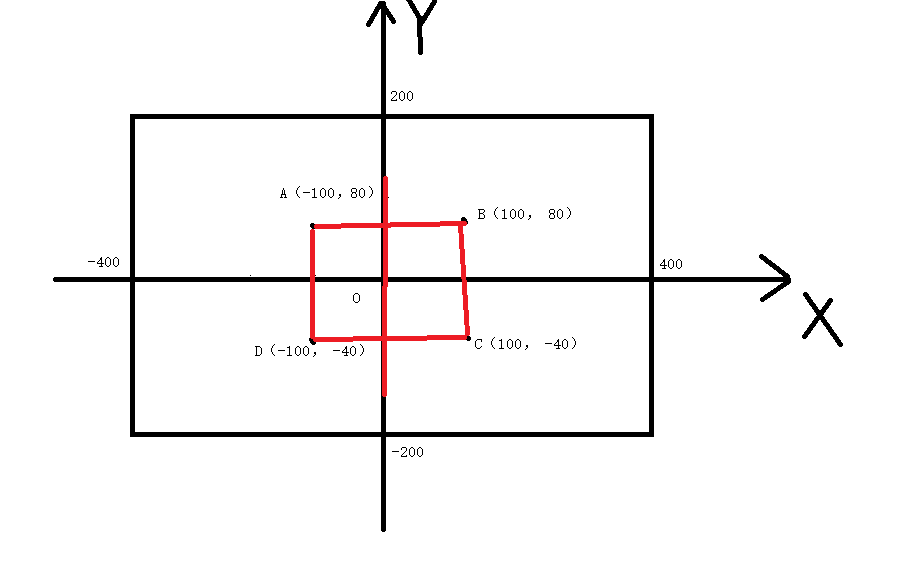然后再连接EF两点。完成。对应的代码：
#zhong
change(-100, 80)#将画笔放在坐标A（-100， 80）
run(0, 200)#从A点画到B点
run(-90, 120)#从B画到C点
run(180, 200)#从C点画到D点
run(90, 120)#从D点画到A点

change(0, 150)#将画笔放在坐标E（0， 150）
run(-90, 300)#从E点画到F点

画自己的名字嘤嘤嘤
import turtle

def run(angle, lenth):
turtle.seth(angle)
turtle.fd(lenth)

def change(x, y):
turtle.penup()
turtle.goto(x, y)
turtle.pendown()
def init():
turtle.pensize(10)
turtle.pencolor("purple")

turtle.setup(800, 400, 200, 200)
init()
#wu
change(-350, 100)
run(0, 100)
run(-90, 70)
run(180, 100)
run(90, 70)
change(-350, 0)
run(0, 100)
change(-400, -50)
run(0, 200)
change(-300, 0)
run(-90, 50)
run(-125, 100)
change(-300, -50)
run(-55, 100)
run(0,10)
#feng
change(-65, 70)
run(0, 130)
change(-50, 10)
run(0, 100)
change(-100, -70)
run(0, 200)
change(0, -160)
run(90, 280)
#yuan
change(125, 100)
run(-35, 40)
change(125, 25)
run(-35, 40)
change(125, -125)
run(55, 90)
change(220, 100)
run(0, 110)
change(220, 100)
run(-95,230)
change(285, 85)
run(-145, 40)
change(250, 65)
run(0, 70)
run(-90, 80)
run(180, 70)
run(90, 80)
change(250, 25)
run(0, 70)
change(285,-15)
run(-90, 120)
run(135, 20)
change(250, -50)
run(-125, 40)
change(315, -50)
run(-55, 50)

展开全文• 我正在学习python学习教程，我无法打开屏幕进行绘图。 我没有发现错误，它只是显示程序完成了运行。 代码如下：import turtle #acutally called turtle to draw a turtle beautiful also used todraw othe...
• 名字白璐璐，请帮忙算坐标，谢谢 import turtle def run(angle, lenth): turtle.seth(angle) turtle.fd(lenth) def change(x, y): turtle.penup() turtle.goto(x, y) turtle.pendown() def init(): turtle.pensize...有问必答
• 我正在学习python学习教程，我无法打开屏幕进行绘图。 我没有发现错误，它只是显示程序完成了运行。 代码如下：import turtle #acutally called turtle to draw a turtle beautiful also used todraw othe...
• ## 用python写名字

千次阅读 2021-05-20 16:08:51
一笔一自己写出来，感觉良好 很简单的操作，记录一下这个特殊日子 代码如下： import turtle from turtle import * def run(angle, lenth): turtle.seth(angle) turtle.fd(lenth) def change(x, y): turtle...
• #1-2心形圆弧 def hart_arc(): for i in range(200): turtle.right(1) turtle.forward(2) def move_pen_position(x, y): turtle.hideturtle() # 隐藏画笔（先） turtle.up() # 提笔 turtle.goto
• 怎么用python画花朵？下面给大家讲解一下具体步骤：第一步，打开菜单栏，输入idle，打开shell。第二步，新建一个文件，并命名。第三步，导入turtle模块，创建一个新窗口用于绘图，再创建一个turtle对象。第四步，...
• 原标题：如何用Python画一只机器猫？本文经授权转自公众号异步图书如若转载请联系原公众号自信心是成功的源泉，对刚入门编程行业的初级程序员来说，多敲代码多做项目就是构建自信心的最好办法。第一次学编程是在屏幕...
• 怎么用python画花朵？下面给大家讲解一下具体步骤：第一步，打开菜单栏，输入idle，打开shell。第二步，新建一个文件，并命名。第三步，导入turtle模块，创建一个新窗口用于绘图，再创建一个turtle对象。第四步，...
• 我正在学习python学习教程，我无法打开屏幕进行绘图。 我没有发现错误，它只是显示程序完成了运行。 代码如下：import turtle #acutally called turtle to draw a turtle beautiful also used todraw ...
• 利用python中的turtle库绘制自己名字 # 利用turtle库绘制自己名字 --杨文博 import turtle as p p.pensize(5) p.pencolor("cornflowerblue") p.setup(1000, 500, 200, 100) p.penup() # 杨 p.goto(-300, 100) p....
• python中常用matplotlib的pie来绘制，基本命令如下所示（python3.X版本）： vals = [1, 2, 3, 4]#创建数据系列 fig, ax = plt.subplots()#创建子图 labels = 'A', 'B', 'C', 'D' colors = ['yellowgreen', 'gold'...
• Python如何函数的曲线输入以下代码导入小编们用到的函数库。 >>> import numpy as np >>> import matplotlib.pyplot as plt >>> x=np.arange(0,5,0.1); >>> y=np.sin(x); plt.plot(x,y) 采用刚才代码后有可能无法...
• 作者 | 人邮异步社区责编 | 胡巍巍出品 | CSDN博客自信心是成功的源泉，对刚入门编程行业的初级程序员来说，多敲代码多做项目就是构建自信心的最好办法。...今天就教大家用Python写一个机器猫，跟着异...
• 本资源为小编原创的使用python绘制的螺旋文字的源代码文件，在文章中小编已经将代码进行了比较详细的注释，有需要的小伙伴们可以下载该资源。
• 在儿童节用Python画一颗童心也不错，关键是，一行代码就可以实现！不说废话，直接上代码： #author: hanshiqiang365 print(' '.join([''.join([('ChildrenDay'[(x-y)%11]if((x*0.05)**2+(y*0.1)**2-1)**3-(x*0.05)...
• 原标题：使用Python的turtle炫酷图形例子一：import turtlet = turtle.Pen()turtle.bgcolor("black")sides=6colors=["red","yellow","green","blue","...
• 一起来玩吧 #五星红旗 import turtle as t t.setup() t.penup() t.goto(-240,160) t.pendown() t.pensize(0.01) t.pencolor('white') #红布 t.fillcolor('red') t.begin_fill() for i in range(2): ...t.pe
• 今天小编就为大家分享一篇python绘制多个子图的实例，具有很好的参考价值，希望对大家有所帮助。一起跟随小编过来看看吧
• ## 用python画美国地图

千次阅读 2020-04-24 09:47:29
• 论文中出各种绚烂的插图，都是一些什么样的软件出来的。本文介绍 Python 的绘图模块 matplotlib: Python plotting。适用于从 2D 到 3D，从标量到矢量的各种绘图。能够保存成从 eps, pdf 到 svg, png, jpg 的...
• ## Python画好看的星空图

万次阅读 多人点赞 2020-04-06 11:39:43
用Python中的turtle库绘制3D星空图。...

# 用python画自己的名字python 订阅